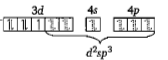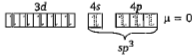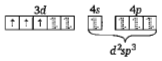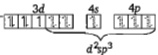Which of the following complex compound(s) is/are paramagnetic and low spin?

(a) ${\mathrm{K}}_{3}\left[\mathrm{Fe}\left(\mathrm{CN}{\right)}_{6}\right]$                       (b) ${\left[\mathrm{Ni}\left(\mathrm{CO}{\right)}_{4}\right]}^{0}$

(c) ${\left[\mathrm{Cr}\left({\mathrm{NH}}_{3}{\right)}_{6}\right]}^{3+}$                       (d) ${\left[\mathrm{Mn}\left(\mathrm{CN}{\right)}_{6}\right]}^{4-}$

Choose the correct code :

(a) I only       (b) II and III        (c) I and IV       (d) IV only

Concept Videos :-

#23 | VBT & CFT: I
#24 | VBT & CFT: II
#25 | VBT & CFT: III
#26 | Colour of Coordination Compounds

Concept Questions :-

VBT & CFT, Limitation /Hybridisation

(c)$⇒$ low spin complex$\mathrm{\mu }=0$$⇒$ low and high spin complex is applicable for ${\mathrm{d}}^{4}$ to ${\mathrm{d}}^{7}$ configurationDifficulty Level:

• 16%
• 27%
• 49%
• 10%